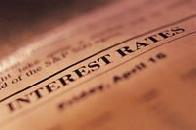Financial Terms Customary payout ratios

# Definition of Customary payout ratios## Customary payout ratios

A range of payout ratios that is typical based on an analysis of comparable firms.

# Related Terms:

## Asset activity ratios

ratios that measure how effectively the firm is managing its assets.

## Capitalization ratios

Also called financial leverage ratios, these ratios compare debt to total capitalization
and thus reflect the extent to which a corporation is trading on its equity. Capitalization ratios can be
interpreted only in the context of the stability of industry and company earnings and cash flow.

## Common stock ratios

ratios that are designed to measure the relative claims of stockholders to earnings
(cash flow per share), and equity (book value per share) of a firm.

## Coverage ratios

ratios used to test the adequacy of cash flows generated through earnings for purposes of
meeting debt and lease obligations, including the interest coverage ratio and the fixed charge coverage ratio.

## Dividend payout ratio

Percentage of earnings paid out as dividends.

## dividend payout ratio

Computed by dividing cash dividends for the year
by the net income for the year. It’s simply the percent of net income distributed
as cash dividends for the year.

## dividend payout ratio

Percentage of earnings paid out as dividends.## Feasible target payout ratios

payout ratios that are consistent with the availability of excess funds to make
cash dividend payments.

## Financial leverage ratios

Related: capitalization ratios.

## Full-payout lease

See: financial lease.

## Leverage ratios

Measures of the relative contribution of stockholders and creditors, and of the firm's ability
to pay financing charges. Value of firm's debt to the total value of the firm.

## Liquidity ratios

ratios that measure a firm's ability to meet its short-term financial obligations on time.

## Liquidity ratios

ratios that measure a firm's ability to meet its short-term financial obligations on time.

## Market value ratios

ratios that relate the market price of the firm's common stock to selected financial
statement items.

## Payout ratio

Generally, the proportion of earnings paid out to the common stockholders as cash dividends.
More specifically, the firm's cash dividend divided by the firm's earnings in the same reporting period.

## payout ratio

Fraction of earnings paid out as dividends.## profit ratios

ratios based on sales revenue for a period. A measure of
profit is divided by sales revenue to compute a profit ratio. For example,
gross margin is divided by sales revenue to compute the gross margin
profit ratio. Dividing bottom-line profit (net income) by sales revenue
gives the profit ratio that is generally called return on sales.

## Profitability ratios

ratios that focus on the profitability of the firm. Profit margins measure performance
with relation to sales. Rate of return ratios measure performance relative to some measure of size of the
investment.

## Rate of return ratios

ratios that are designed to measure the profitability of the firm in relation to various
measures of the funds invested in the firm.

## Reserve ratios

Specified percentages of deposits, established by the Federal Reserve Board, that banks must
keep in a non-interest-bearing account at one of the twelve Federal Reserve Banks.

## Short-term solvency ratios

ratios used to judge the adequacy of liquid assets for meeting short-term
obligations as they come due, including
1) the current ratio,
2) the acid-test ratio,
3) the inventory turnover ratio, and
4) the accounts receivable turnover ratio.

## Target payout ratio

A firm's long-run dividend-to-earnings ratio. The firm's policy is to attempt to pay out a
certain percentage of earnings, but it pays a stated dollar dividend and adjusts it to the target as base-line
increases in earnings occur.